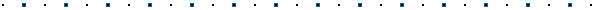#Written by Robert Dunlop Microsoft DirectX MVP## Introduction

When using programmable vertex shaders in DirectX Graphics, sample implementations usually rely on transposing transformation matrices prior to setting them as shader constants.  This is because the transformation macros or their equivalent instructions are geared toward column major matrices, while Direct3D's fixed function pipeline requires row major matrices.  In this article we will take a quick look at how to perform vertex shader transformations using row major matrices, avoiding the need to transpose existing matrices.

## First, a look at using transposed matrices

The vertex shader language provides us with a macro, m4x4, that encapsulates 4x4 matrix transformation of a 4D vector.  This macro expands to four dp4 commands, taking dot products of the vector being transformed with each of 4 sequential constant registers, each dp4 command generating one component of the result:

 m4x4 r0, v0, c1 equates to dp4 r0.x, v0, c1 dp4 r0.y, v0, c2 dp4 r0.z, v0, c3 dp4 r0.w, v0, c4

This assumes that each 4x4 vector represents the transformation of the input vector to a single component of the output vector.  When we use SetVertexShaderConstant() to set the constants to an input matrix, the result is four 4D vectors each representing a row from the matrix.  This requires the matrix to be column-major.  However, the matrices used to set up the fixed function pipeline are  row-major, as are any matrices generated using the D3DX helper functions.  Thus, to use this method we must first transpose any matrices we use before applying them as a shader constant:

pDev->SetTransform(&m_worldMat);              // set fixed function transformation
D3DXMATRIX tempMat;
D3DXMatrixTranspose(&tempMat,&m_worldMat);    // transpose the matrix

## Using Row-Major Matrices in a Shader

It is possible, however, to use the same row-major matrices we use for the fixed function pipeline in our shaders, though, at no added cost.  All we do is change to a summation of four vector products, rather than summing the result of each product to for a single component.  The method for transforming with row-major matrices looks like this:

 mul r0, v0, c1 mad r0, v0, c2, r0 mad r0, v0, c3, r0 mad r0, v0, c4, r0

This allows us to use the same matrices for the fixed pipeline and for our programmable vertex shaders:

pDev->SetTransform(&m_worldMat);                // set fixed function transformationThis site, created by DirectX MVP Robert Dunlop and aided by the work of other volunteers, provides a free on-line resource for DirectX programmers.

Special thanks to WWW.MVPS.ORG, for providing a permanent home for this site.

#####Visitors Since 1/1/2000:Last updated: 07/26/05.